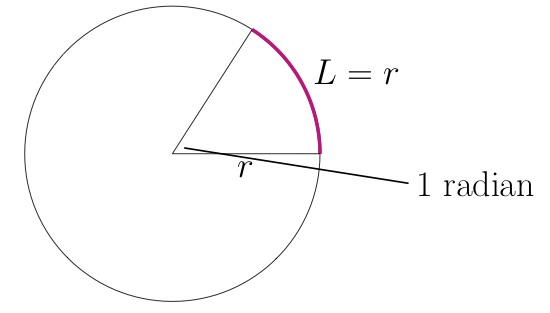# Arc Length and Central Angle

## Problem

Pictured is a circle with central angle x, radius 100, and an arc whose length issomewhere between 70 and 90.  What are the possible values, in degrees, of x?

## Solution

An arc is a fraction of a circumference. What fraction? An arc’s length is the same proportion of a circle’s circumference as its central angle is of a whole circle. And a circle’s circumference equals 2π times the circle’s radius.

Then , where L is arc length and x is a central angle, so .

Consider the extremes of the range you’re given:  and  What is x for those values of arc length?

 For L=70: For L=90:

So x measures between 40.1 and 51.6 degrees.

Mini-lesson: What’s a radian?